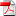# Deep neural networks algorithms for stochastic control problems on finite horizon, part I : convergence analysis - C. Hure, H. Pham, A. Bachouch and N. Langrené

18
Jan

This paper develops algorithms for high-dimensional stochastic control problems based on deep learning and dynamic programming (DP). Diffrently from the classical approximate DP approach, we rst approximate the optimal policy by means of neural networks in the spirit of deep reinforcement learning, and then the value function by Monte Carlo regression. This is achieved in the DP recursion by performance or hybrid iteration, and regress now or later/quantization methods from numerical probabilities. We provide a theoretical justi cation of these algorithms. Consistency and rate of convergence for the control and value function estimates are analyzed and expressed in terms of the universal approximation error of the neural networks. Numerical results
on various applications are presented in a companion paper  and illustrate the performance of our algorithms.

•RR-FIME-18-10